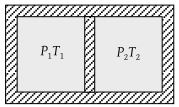NEET Physics Thermodynamics Questions Solved

Following figure shows on adiabatic cylindrical container of volume V0 divided by an adiabatic smooth piston (area of cross-section = A) in two equal parts. An ideal gas $\left({C}_{P}/{C}_{V}=\gamma \right)$ is at pressure P1 and temperature T1 in left part and gas at pressure P2 and temperature T2 in right part. The piston is slowly displaced and released at a position where it can stay in equilibrium. The final pressure of the two parts will be (Suppose x = displacement of the piston)(1) P2

(2) P1

(3) $\frac{{P}_{1}{\left(\frac{{V}_{0}}{2}\right)}^{\gamma }}{{\left(\frac{{V}_{0}}{2}+Ax\right)}^{\gamma }}$

(4) $\frac{{P}_{2}{\left(\frac{{V}_{0}}{2}\right)}^{\gamma }}{{\left(\frac{{V}_{0}}{2}+Ax\right)}^{\gamma }}$

Concept Videos :-

#3 | Thermodynamic Processes
#4 | Isobaric Process
#5 | Isochoric & Isothermal Process
#6 | Interconversion of Graphs
#7 | Solved Example: 1
#8 | Solved Example: 2
#9 | Adiabatic Process
#10 | Solved Example: 3
#11 | Graphs in Adiabatic Process: 1
#12 | Graphs in Adiabatic Process: 2

Concept Questions :-

Types of processes
Explanation is a part of a Paid Course. To view Explanation Please buy the course.

Difficulty Level: